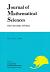## Vol. 13 (2006) No. 02Journal of Mathematical Sciences The University of Tokyo

1. Minakawa, Hiroyuki
Examples of pseudo-Anosov homeomorphisms with small dilatations
Vol. 13 (2006), No. 2, Page 95--111.

2. Koshimizu, Hiroshi
An Extension Theorem for Real Analytic Solutions to Relative Hyperbolic Systems
Vol. 13 (2006), No. 2, Page 113--127.

3. Otobe, Yoshiki
Stochastic Partial Differential Equations with Two Reflecting Walls
Vol. 13 (2006), No. 2, Page 129--144.
Studies on the Painlev\'e Equations, V, Third Painlev\'e Equations of Special Type $P_{\rm III}(D_7)$ and $P_{\rm III}(D_8)$